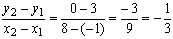# Slope of Linear Functions

The concept of slope is important in economics because it is used to measure the rate at which changes are taking place. Economists often look at how things change and about how one item changes in response to a change in another item.

It may show for example how demand changes when price changes or how consumption changes when income changes or how quickly sales are growing.

Slope measures the rate of change in the dependent variable as the independent variable changes. The greater the slope the steeper the line.

Consider the linear function:

y = a + bx

b is the slope of the line. Slope means that a unit change in x, the independent variable will result in a change in y by the amount of b.

slope = change in y/change in x = rise/run

Slope shows both steepness and direction. With positive slope the line moves upward when going from left to right. With negative slope the line moves down when going from left to right.

If two linear functions have the same slope they are parallel.

Slopes of linear functions

The slope of a linear function is the same no matter where on the line it is measured. (This is not true for non-linear functions.)An example of the use of slope in economics

Demand might be represented by a linear demand function such as

Q(d) = a - bP

Q(d) represents the demand for a good

P represents the price of that good.

Economists might consider how sensitive demand is to a change in price.This is a typical downward sloping demand curve which says that demand declines as price rises.This is a special case of a horizontal demand curve which says at any price above P* demand drops to zero. An example might be a competitor's product which is considered just as good.This is a special case of a vertical demand curve which says that regardless of the price quantity demanded is the same. An example might be medicine as long as the price does not exceed what the consumer can afford.

Supply might be represented by a linear supply function such as

Q(s) = a + bP

Q(s) represents the supply for a good

P represents the price of that good.

Economists might consider how sensitive supply is to a change in price.This is a typical upward sloping supply curve which says that supply rises as price rises.

An example of the use of slope in economics

The demand for a breakfast cereal can be represented by the following equation where p is the price per box in dollars:

d = 12,000 - 1,500 p

This means that for every increase of \$1 in the price per box, demand decreases by 1,500 boxes.

Calculating the slope of a linear function

Slope measures the rate of change in the dependent variable as the independent variable changes. Mathematicians and economists often use the Greek capital letter D or D as the symbol for change. Slope shows the change in y or the change on the vertical axis versus the change in x or the change on the horizontal axis. It can be measured as the ratio of any two values of y versus any two values of x.Example 1

Find the slope of the line segment connecting the following points:

(1,1) and (2,4)

x1 = 1              y1 = 1

x2 = 2              y2 = 4Example 2

Find the slope of the line segment connecting the following points:

(-1,-2) and (1,6)

x1 = -1              y1 = -2

x2 = 1               y2 = 6Example 3

Find the slope of the line segment connecting the following points:

(-1,3) and (8,0)

x1 = -1              y1 = 3

x2 = 8               y2 = 0[Index]